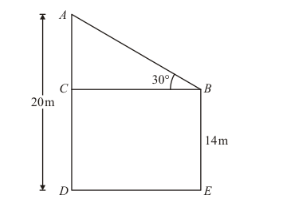# The tops of two poles of height 20 m and 14 m are connected

Question:

The tops of two poles of height 20 m and 14 m are connected by a wire. If the wire makes an angle of 30° with horizontal, then the length of the wire is

(a) 12 m

(b) 10 m

(c) 8 m

(d) 6 m

Solution:

Letbe the length of wire.Given that wire makes an angleNow, $A C=(20-14) \mathrm{cm}=6 \mathrm{~cm}$,

Here, we have to find length of wire.

So we use trigonometric ratios.

In a triangle,

$\Rightarrow \sin B=\frac{A C}{A B}$'

$\Rightarrow \sin 30^{\circ}=\frac{6}{h}$

$\Rightarrow \frac{1}{2}=\frac{6}{h}$

$\Rightarrow h=12$

Hence the correct option is a